# allrite

## Grooving of a Bicrystal – Derivation of the Evolution Equation

We consider a surface with only one non-zero principal curvature, given by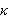. The chemical potential of the surface is given by: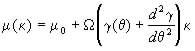(1)

(Herring, 1951) where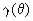is the surface tension along the crystalline orientation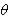and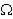is the atomic volume. The chemical potential at zero curvature is given by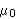and that lattice vacancies are in equilibrium and thus contribute no chemical potential.

The flux, J, due to surface diffusion is given by the Nerst-Einstein relation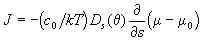(2)

which, after substituting (1) becomes: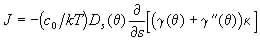. (3)

Here,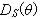is the surface diffusion and s is the arc length. The first set of symbols are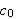, the number of atoms per unit area in one monolayer, k, the Boltzmann constant and T the temperature, are all considered constant.

Taking the negative divergence of J and multiplying by the atomic volume the normal velocity due to surface diffusion is obtained.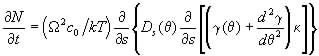(4)

If the surface profile is denoted by y(x,t) then equation (4) in Cartesian coordinates becomes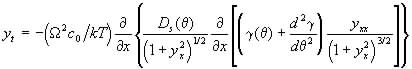(5)

Hereis chosen to be the angle from the y axis to the normal of the surface and can thus be given as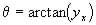.